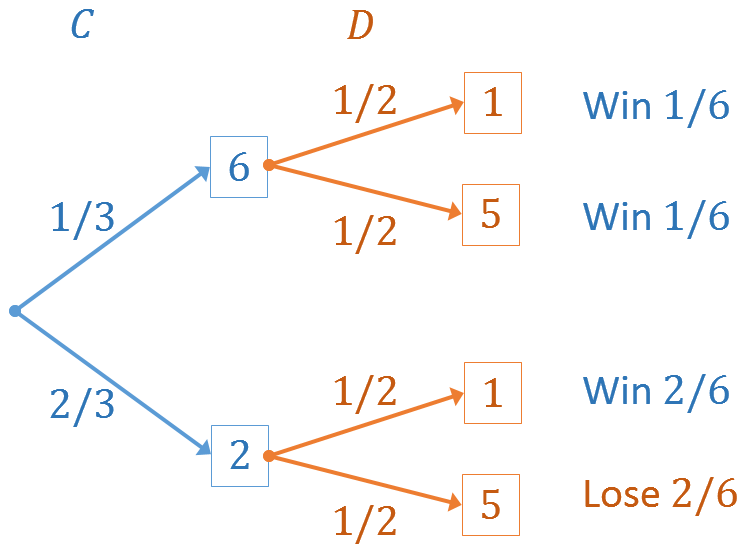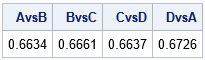Most games of skill are transitive. If Player A wins against Player B and Player B wins against Player C, then you expect Player A to win against Player C, should they play. Because of this, you can rank the players: A > B > C

Interestingly, not all games are transitive. The game "Rock, Paper, Scissors," is an intransitive game. In this game, Rock beats Scissors, Scissors beats Paper, and Paper beats Rock. You cannot rank the game shapes. You cannot declare that one shape wins more often than another.

In "Rock, Paper, Scissors," both players display their shapes simultaneously. If not, the second player could win 100% of the time by knowing the shape that is shown by the first player.

### Intransitive dice

Recently, I saw an intransitive game that involves special six-sided dice. In this game, there are four dice: A, B, C, and D. Two players each choose a die and roll it. The higher number wins. If the players choose their die sequentially, the second player is at a huge advantage: No matter which die the first player chooses, the second player can choose a die such that his probability of winning the game is 2/3.

Clearly, the numbers on the faces of the dice are important since this advantage does not exist for the usual six-sided dice. One set of intransitive dice have faces as follows:
A = {0, 0, 4, 4, 4, 4}
B = {3, 3, 3, 3, 3, 3}
C = {2, 2, 2, 2, 6, 6}
D = {1, 1, 1, 5, 5, 5}

For these dice:

• A wins against B with probability 2/3.
• B wins against C with probability 2/3.
• C wins against D with probability 2/3.
• D wins against A with probability 2/3.

The probability of winning for pairs that involve die B are easy to compute because that die has a 3 on every face. By inspection, die A will win against B whenever A shows a 4, which occurs 2/3 of the time. Similarly, die B wins against die C whenever C shows a 2, which is 2/3 of the time.

The remaining probabilities can be computed by using a tree diagram for conditional probability. A tree diagram for the dice C and D are shown below. If C shows a 6 (which happens 1/3 of the time), then C wins. If C shows a 2 (which happens 2/3 of the time), then the game depends on the roll of D. If D rolls a 1 (which happens 1/2 of the time), then C wins. But if D rolls a 5 (which happens 1/2 of the time), then C loses. Since the rolls are independent, you multiply the individual probabilities to obtain the total probability of each pair of events. For C versus D, C wins with probability 2/3.You can construct a similar diagram for the D versus A game. It is also interesting to compute the probability of A versus C and B versus D for these dice.

### Simulate many games

Because conditional probability can be tricky, I always like to check my computations by running a simulation. The following SAS/IML program uses the SAMPLE function to simulate 10,000 rolls of each die. The estimated probability for winning is the number of times that one die showed the greater number, divided by 10,000, which is the total number of simulated games. For each of the four games considered, the estimated probability is approximately 2/3.

```proc iml; /* define the dice */ A = {0, 0, 4, 4, 4, 4}; B = {3, 3, 3, 3, 3, 3}; C = {2, 2, 2, 2, 6, 6}; D = {1, 1, 1, 5, 5, 5};   call randseed(1234); N = 10000; /* simulate N rolls for each die */ sA = sample(A, N); sB = sample(B, N); sC = sample(C, N); sD = sample(D, N);   /* estimate the probability of 'A vs B', 'B vs C', etc */ AvsB = sum(sA > sB) / N; BvsC = sum(sB > sC) / N; CvsD = sum(sC > sD) / N; DvsA = sum(sD > sA) / N; print AvsB BvsC CvsD DvsA;```